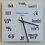# Foo function

Recently I took the test for Computer Science and I got a Level 3... I couldn't figure out the Level 4 Question and I found it quite interesting and could anyone explain me how it works..?

How many '1' s are printed out when you call foo(5)?

   # python code start
def foo(n):
count = 1
if n > 0:
count += foo(n - 1) + foo(n - 1)
print '1'
return countNote by Muzaffar Ahmed
6 years, 7 months ago

This discussion board is a place to discuss our Daily Challenges and the math and science related to those challenges. Explanations are more than just a solution — they should explain the steps and thinking strategies that you used to obtain the solution. Comments should further the discussion of math and science.

When posting on Brilliant:

• Use the emojis to react to an explanation, whether you're congratulating a job well done , or just really confused .
• Ask specific questions about the challenge or the steps in somebody's explanation. Well-posed questions can add a lot to the discussion, but posting "I don't understand!" doesn't help anyone.
• Try to contribute something new to the discussion, whether it is an extension, generalization or other idea related to the challenge.

MarkdownAppears as
*italics* or _italics_ italics
**bold** or __bold__ bold
- bulleted- list
• bulleted
• list
1. numbered2. list
1. numbered
2. list
Note: you must add a full line of space before and after lists for them to show up correctly
paragraph 1paragraph 2

paragraph 1

paragraph 2

[example link](https://brilliant.org)example link
> This is a quote
This is a quote
    # I indented these lines
# 4 spaces, and now they show
# up as a code block.

print "hello world"
# I indented these lines
# 4 spaces, and now they show
# up as a code block.

print "hello world"
MathAppears as
Remember to wrap math in $$ ... $$ or $ ... $ to ensure proper formatting.
2 \times 3 $2 \times 3$
2^{34} $2^{34}$
a_{i-1} $a_{i-1}$
\frac{2}{3} $\frac{2}{3}$
\sqrt{2} $\sqrt{2}$
\sum_{i=1}^3 $\sum_{i=1}^3$
\sin \theta $\sin \theta$
\boxed{123} $\boxed{123}$

Sort by:

:) There's a trick.

- 6 years, 7 months ago

So tell me please :) I know we can directly execute it but I want to understand how it works..

- 6 years, 7 months ago

Have you tried doing it for $n=1$ and $n=2$?

Staff - 6 years, 7 months ago

Nope.. I'm not so good at programming so can you please explain me this one from the beginning..?

- 6 years, 7 months ago

If you call foo(1), it is greater than zero and, so, the function passes the if statement. It the adds 2*foo(0) (which needs to be called) to count and prints 1.

When it calls foo(0), 0 is not greater than 0 and, so, the if statement is not passed. The function then runs the code that appears after the if statement, where it returns the current value of count which is 1 and prints 1. However, foo(0) was called twice (count += foo(0) + foo(0)), so it prints 1 twice.

So, for foo(1), we print 1 a total three times.

If we called foo(2), this same kind of branching process would occur. I.e., we'd print 1, then we'd call foo(1) twice, which would print 1 twice, then for each foo(1), we'd call foo(0) twice (a total of 4 foo(0)) calls, which would print 1 four times. Making a total of 7. In general, calling foo(n), we print 1 a total of $2^{n+1}-1$ times.

Staff - 6 years, 7 months ago

Ohh.. I got it.. ! Thanks bro :-)

- 6 years, 7 months ago

No problem. It was foon to explain.

Staff - 6 years, 7 months ago

Lol :D

- 6 years, 7 months ago